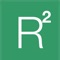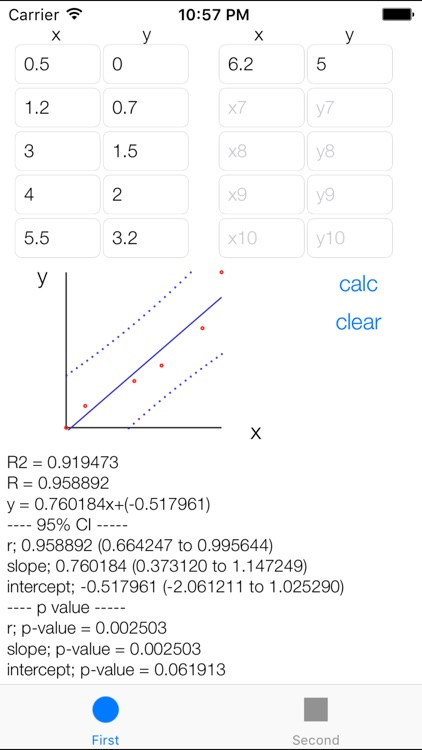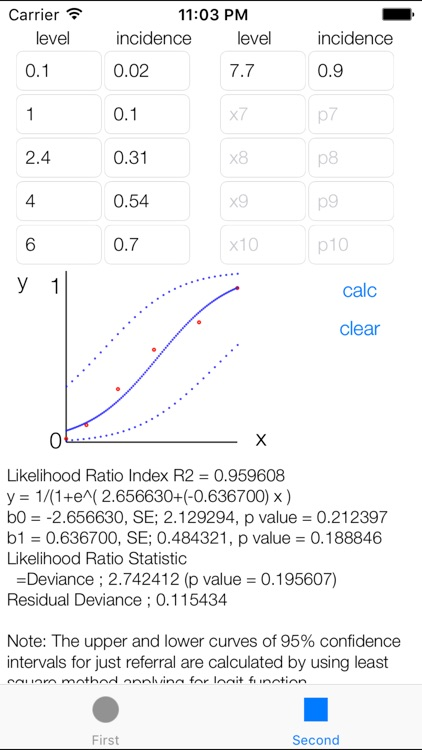## This statistical analysis app for at most ten pairs of data in terms of linear regression analysis or logistic regression analysis# L-Regression

by Yasunari Miyagi, MDThis statistical analysis app for at most ten pairs of data in terms of linear regression analysis or logistic regression analysis. Not only parameters but also regression line with 95% confidence intervals are presented.### App Details

Version
1.6
Rating
NA
Size
4Mb
Genre
Medical Education
Last updated
January 15, 2019
Release date
February 10, 2012

### App Store Description

This statistical analysis app for at most ten pairs of data in terms of linear regression analysis or logistic regression analysis. Not only parameters but also regression line with 95% confidence intervals are presented.

1. Linear regression anlaysis.
Regression formula is presented. R2, 95% confidence intervals, with p-values are calculated.

2. Logistic regression analysis.
Regression formula is presented. Likelihood ratio index (R2), coefficients with p-values, Likelihood Ratio Statistic (= Deviance) with p-value, Residual Deviance are calculated.
The upper and lower 95% CI for referral, calculated by using least square method applying for logit function.

Disclaimer:
AppAdvice does not own this application and only provides images and links contained in the iTunes Search API, to help our users find the best apps to download. If you are the developer of this app and would like your information removed, please send a request to [email protected] and your information will be removed.